## 3.9.3 Current Density

To derive an equation for the current density one uses the conservation law of quantum mechanical variables . The starting point is the subtraction of equation (3.62) from (3.63)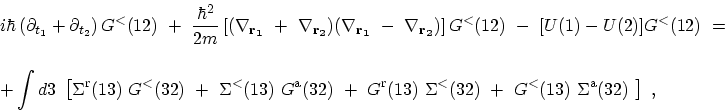(3.85)

where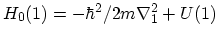has been assumed. By taking the limit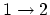(and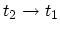) and assuming that the right-hand-side of (3.85) approaches zero in this limit, one obtains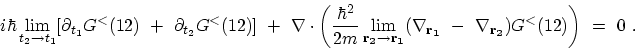(3.86)

By multiplying both sides by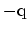and recalling the definition of the charge density, one recovers the continuity equation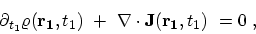(3.87)

where the current density is defined as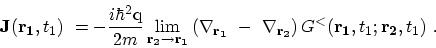(3.88)

Under steady-state condition the current density takes the form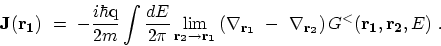(3.89)

M. Pourfath: Numerical Study of Quantum Transport in Carbon Nanotube-Based Transistors